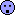## Now what? w & w* examples [Two-Stage / GS Designs]

Dear Helmut,

I have tried to demystify some aspects of choosing w and w* for the maximum combination test by looking into some examples:

Take nfix as sample size in stage 1 (Helmut’s proposal)
Guess:
CV=0.2, theta0=0.95 -> nfix = 20
Choose n1 = nfix = 20, i.e. w= 0.99, since w has to be <1.

Guess was too pessimistic:
e.g. true CV=0.15 -> nfix = 12
or theta0=0.975 -> nfix = 16
For both the sample size for stage 1 exceed the necessary total sample size of a fixed design. Thus a more realistic w* can’t be defined or should be set to the same value as w.
This results in the standard combination test.

Guess was too optimistic:
e.g. true CV=0.25 -> nfix = 28
or theta0=0.925 -> nfix = 26
Both lead to a ‘more realistic’ w*= 0.71 or 0.77. Let's choose w* = 0.7 for simplicity.

Power & sample size of the scenarios
                                                                N                                                        ------------------                    CV   theta0  w      w*     power    ASN   Median  p95% ------------------------------------------------------------------------- Guess             0.20  0.95   0.99   0.5*)   0.866    21.5    20     34                                0.99   0.99    0.872    24.9    20     30                                0.99   0.7     0.870    21.5    20     28 Too pessimistic   0.15  0.95   0.99   0.99    0.966    20.1    20     20                   0.20  0.975  0.99   0.99    0.936    22.9    20     24 Too optimistic    0.25  0.95   0.99   0.7     0.842    29.1    20     64                   0.20  0.925  0.99   0.7     0.760    22.6    20     36 ------------------------------------------------------------------------- *) w* = w/2 according to Maurer et al. No futility criterion

Take nfix/2 as sample size in stage 1 (Maurer et al.)
Guess:
CV=0.2, theta0=0.95 -> nfix = 20
Choose n1 = nfix/2 = 10, i.e. w= 0.5.

Guess was too pessimistic:
e.g. true CV=0.15 -> nfix = 12
or theta0=0.975 -> nfix = 16
This would let to a ‘more realistic’ w*= 0.83 or 0.625, respectively. Let's take for simplicity w* = 0.7.

Guess was too optimistic:
e.g. true CV=0.25 -> nfix = 28
or theta0=0.925 -> nfix = 26
Both lead to a ‘more realistic’ w*= 0.36 or 0.38. Let's take for simplicity w* = 0.4.

Power & sample size of the scenarios
                                                                N                                                        ------------------                    CV   theta0  w      w*     power    ASN   Median  p95% ------------------------------------------------------------------------- Guess             0.20  0.95   0.5    0.25*)  0.838    22.7    20     46                                0.5    0.7     0.844    22.6    18     50                                0.5    0.4     0.841    22.5    20     48 Too pessimistic   0.15  0.95   0.5    0.7     0.881    13.0    10     24                   0.20  0.975  0.5    0.7     0.896    21.4    18     48 Too optimistic    0.25  0.95   0.5    0.4     0.822    37.1    34     78                   0.20  0.925  0.99   0.4     0.747    24.1    20     52 ------------------------------------------------------------------------- *) w* = w/2 according to Maurer et al. No futility criterion

Confusion:
• Different weights w* don’t make a big difference, I think
• Too pessimistic specifications result in higher power and lower expected sample size (!)(at least for CVs around 0.2)
• Too optimistic specifications may result in lower power and higher expected sample size (!)
• Choosing the sample size for stage 1 as the sample size of a fixed design seems to have some advantages w.r.t. power and expected sample size compared to the 'midterm' sample size re-estimation. Except the too pessimistic settings row CV=0.15, theta0=0.95 ... for the 'midterm' SSR.

Regards,

DetlewIng. Helmut Schütz